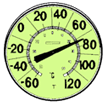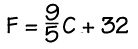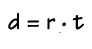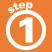Home    |    Teacher    |    Parents    |    Glossary    |    About UsFormulas are equations that state a fact or a rule relating two or more variables. You can solve a formula for any of its variables using the rules for solving equations.

Here are two commonly used formulas. Click each one to see how to solve it.Homework Help | Algebra | Equations and InequalitiesEmail this page to a friendSearch·  Solving addition and     subtraction equations·  Multiplication equations·  Division equations·  Inequalities·  Formulas·  Two-step equations     and inequalitiesFirst Glance In Depth Examples WorkoutInequalities